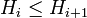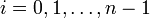# Supercharacter theory corresponding to a normal series

## Definition

Suppose$G$ is a finite group. Consider a normal series for$G$, i.e., groups:$\{ e \} = H_0 \le H_1 \le \dots \le H_n = G$

such that each$H_i$ is a normal subgroup of$G$. The supercharacter theory corresponding to this normal series is a supercharacter theory defined as follows:

### Blocks of conjugacy classes

There are$n + 1$ blocks of conjugacy classes, i.e., there are$n + 1$ superconjugacy classes. As sets of elements, these are the set differences:$H_0, H_1 \setminus H_0, H_2 \setminus H_1, \dots, H_n \setminus H_{n-1}$

As blocks of conjugacy classes, the block corresponding to each is just the set of conjugacy classes in it.

### Blocks of representations and corresponding supercharacters

The blocks of linear representations are as follows. There are$n + 1$ blocks. The block corresponding to the containment$H_i \le H_{i + 1}$ is the set of those irreducible linear representations whose kernel contains$H_i$ but not$H_{i+1}$. In addition to these$n$ blocks for$i = 0,1,\dots,n-1$, there is the trivial representation, which forms its own block.

We can choose the supercharacters as follow. For the trivial representation, the supercharacter is the trivial character itself. For a containment$H_i \le H_{i+1}$, the supercharacter is:

(Character of the regular representation of$G/H_i$ composed with the quotient map) - (Character of the regular representation of$G/H_{i+1}$ composed with the quotient map)

Alternatively, this supercharacter is a weighted sum of the characters in the corresponding block with the weight on a character equal to its degree.